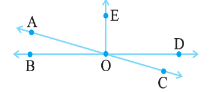# 14.  In the adjoining figure, name the following pairs of angles.(i) Obtuse vertically opposite angles                                      (ii) Adjacent complementary angles (iii) Equal supplementary angles (iv) Unequal supplementary angles (v) Adjacent angles that do not form a linear pair(i)   are the vertically obtuse angles.

(ii)   are the complementary angles.

(iii)   are the equal supplementary angles.

(iv)   are the unequal pair of supplementary angle.

(v)  ,   and   are adjacent angles but are not supplementary angles.

Exams
Articles
Questions